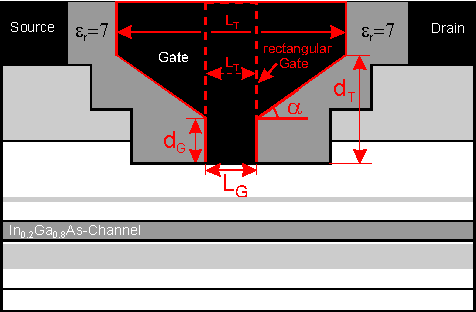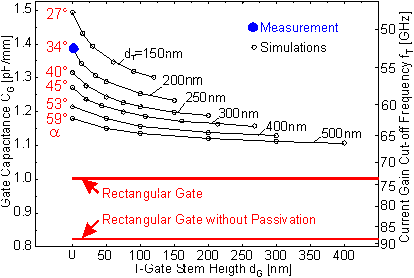Next: 6.3 Millimeter Wave HEMTs Up: 6.2.3 RF Characteristics Previous: 6.2.3.3 Dependence of CG on Passivation Thickness

6.2.3.4 Dependence of CG on T-Gate Cross Section

To investigate the influence of the gate cross section, different gate geometries were simulated by varying the two characteristic dimensions dG and dT. All calculations were performed for LG = 220 nm and LT = 820 nm except for the case of a rectangular gate as depicted in  Figure 6.36. One limiting case for the gate geometry is dG = 0 (as shown in  Figure 6.33 and used for all simulations in Section 6.2 discussed up to here) whereas the other extreme is given by dG = dT ("ideal" T­gate cross section).Figure 6.36 Parameters to investigate the influence of the gate cross section on CG.

Figure 6.37 presents simulations of CG and fT as a function of dG for a completely passivated device with dT as a parameter. The closed circle in  Figure 6.37 designates measurement and simulation of the particular HEMT shown in  Figure 6.33. Here, a distinct T­gate stem does not exist (dG = 0). According to  Figure 6.37, the fT of this device could be increased from 53 GHz to a value close to 60 GHz if the gate cross section could be improved in a way that dG changes from zero to 200 nm (dG = dT, ideal T­gate). Further increase of dG and dT would further improve fT. Values of about 65 GHz are expected for dG = dT = 400 nm, without decreasing the gate length. In the case that dG = 0 and only dT = 400 nm (gate stem sidewalls and device surface enclose an angle of 53°), again fT  60 GHz is expected, comparable to the case dG = dT = 200 nm.Figure 6.37 Simulated gate capacitance CG and current gain cut-off frequency fT for LG = 220 nm and different gate cross sections (VDS = 2.0 V, VGS = 0.4 V). All calculations for LT = 800 nm.

Thus, it is of immense importance that the process technology is able to realize a gate cross section where the gate stem sidewalls are really perpendicular to the semiconductor surface (ideal T­gate). If the enclosed angle is significantly smaller than 90° (V­shaped gate stem), dT has to be substantially greater compared to the perpendicular case if the same fT has to be realized. The multi-resist level approach of most EBL processes facilitates the fabrication of such vertical T­gate stems as shown in  Figure 6.3. Gate technologies that use optical lithography are usually based on narrowing the comparatively large resist openings by spacers. They often have the property not to result in really vertical sidewalls as illustrated in  Figure 6.2. If such a technology is used for the fabrication of devices for high-frequency application, it is important that at least the part of the gate immediately adjacent to the semiconductor surface has perpendicular sidewalls.

A rectangular gate shape shown in  Figure 6.36 represents the limit for arbitrarily increased dT and dG. The CG of a fully passivated device with a rectangular gate is indicated in  Figure 6.37 by the upper bold line and the CG for a device without passivation by the lower bold line.

The upper limit in fT is marked by the case of a rectangular gate and no passivation which decreases the total CG to about 820 fF/mm and increases fT to 88 GHz. Both rectangular gate and no passivation are rather unpractical limits although they can be realized as reported in . The disadvantage of a rectangular gate is a significant increase in the gate resistance RG. This becomes especially important for devices with long gate fingers such as power devices and for extremely high frequencies of up to 100 GHz were the skin effect becomes significant.

Based on the results presented so far the following considerations on the optimization of the RF performance of the HEMT investigated here can be made. A reduction of the gate length from 220 nm to 120 nm would reduce CG by 320 fF/mm. The drawback would be a larger g0. As shown in Section 6.1.2 for the single heterojunction HEMT g0 increases by 100 % to 30 mS/mm which is still an acceptable value. In addition a single heterojunction HEMT is a worst case assumption because it is expected that short channel effects are more severe due to the lower barrier height under the channel compared to a double heterojunction HEMT.

A realistic optimization of the T­gate shape with dG = 150 nm and dT = 300 nm would yield a reduction of CG of 210 fF/mm. This reduces A2 of (62) from 85 fF/mm to about 55 fF/mm such that a reduction of the passivation thickness would have a reduced impact. For a realistic reduction from 700 nm to a residual passivation thickness of 200 nm a reduction in CG by about 70 fF/mm can be expected. These improvements together sum up to a reduction CG from 1.38 pF/mm to about 0.8 pF/mm which translates into fT = 92 GHz.

This shows that to practically increase fT to significantly over 100 GHz a higher gm is necessary. This can be achieved in first place by a smaller gate-to-channel separation. Unfortunately this goes along with an increase in CG due to an increase in A3 of (62). But even if the quotient of gm/A3 would remain constant this would still increase fT due to the parasitic capacitance which are unchanged by a reduction of dGC. A detailed investigation on the optimization of the RF performance of double heterojunction HEMTs will be given in the following section.Next: 6.3 Millimeter Wave HEMTs Up: 6.2.3 RF Characteristics Previous: 6.2.3.3 Dependence of CG on Passivation Thickness

Helmut Brech
1998-03-11